#### Something in Common Rich TasksThis page contains a truly wonderful set of activities created and kindly shared by John Burke. They allow consolidation of key skills, prevent students from copying each other (as all the questions are different), make marking and assessing easy for the teacher (as all the answers are the same!), and provide a lovely extra challenge for students as they try to figure out exactly what is going on! In short, I think they are amazing, and thank you so much to John for sharing them with us all.

Please note: the "Earliest Year Group" is just a guideline. I have often been able to use a "Year 8 Task" with my Year 11s, for example.

#### Contents

This set of resources came into being from my desire, as a teacher, to get pupils to think for themselves and not copy work from their neighbours.  I wish I could say that I did all the research and came up with this scheme but, in fact, it was serendipitous.  I put together a task for some sixth formers (SIC_6 "Annulus Volume of Revolution") where they all had different input parameters but all had the same answer, using a result I remembered from my sixth form days.  It went very well so I developed more resources for lower down the school.  As the pupils caught on there was the risk that they would just sit back and await the answer so one ruse I use is to give each worksheet a letter and say that I have a table of answers that I look up.  Then I introduced some activities where the answers are different but can easily be computed from the input parameters (if you know how) to avoid this issue.  A good example of this is SIC_11 "Triangle xy Area" where the teacher only has to multiply two integers together to know the answer but the pupils don't know that - yet.  I started off labelling my resources "Different Problem, Same Answer!" but that didn't really fit in the end so I ended up with "Something In Common".

Not all of the resources are mine.  Like all teachers I take inspiration from wherever I find it and so have included, for example, some RISPs by Jonny Griffiths and tasks from the nRich website and other places and I give due credit for a task where I remember where it came from.

Each resource has a suggested earliest year group.  This is solely for use by the Mathematics department at my school so that we can avoid the situation whereby a teacher has planned to use a resource and then find that some pupils say they have already completed the task in a previous year.  Please use a resource wherever you see fit.  Indeed, the earliest year group can be a bit misleading because although the scheme of work indicates that the knowledge to perform the task is imparted to that year group the maturity of their problem solving capabilities may not be up to it and so would need a lot of scaffolding anyway.  So, in a way, you can ignore the earliest year group information!  Just review the activity and use it where you see fit.

Because the worksheets are all different I have placed them in pdf format with 1-up, 2-up or 4-up printing already prepared.  All you need do is print the appropriate number of pages for the size of your class.  Every accompanying Powerpoint has a worked solution and the original worksheets can be found at the back of it (so you can create separate ones for visually impaired pupils, for example, or change wording).  Some activities have additional resources like Geogebra files for demonstration.  One even has a Scratch program!

I use the resources in different ways.  Sometimes I use them to introduce a topic (circle theorems and sine rule, for example), sometimes to consolidate the learning of a topic and sometimes to introduce a wow factor on a Friday.  But in every case I encourage/challenge them to find out how I did it and quite often they are up for the challenge, which I find very satisfying, and I hope they will tell their friends.
I enjoy creating these resources and as of October 2016 there are 50 in the set.  They do take time but I and my colleagues at school reuse them a lot and I hope you will too.  As a result I would be grateful for any feedback, whether it is about mistakes or suggestions for improvement or indeed new ideas for a resource.

John Burke
(The Manchester Grammar School)
[email protected]Get pupils to draw a quadrilateral that they think will not tessellate.  Then reproduce it using the Geogebra file and show that it does.  Then show a picture proof.

Worksheet PowerPoint Supporting Materials

###### 15. Magic FractionsnRich.  Using their own numbers (following a rule) the pupils form fractions and carry out operations on them.  All answers are the same.

Worksheet PowerPoint Supporting Materials
None Magic Fractions None

###### 1. Think of a Number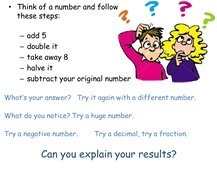Simple game that finishes on 1, whatever your starting number.  Leads to creating expressions and proof.

Worksheet PowerPoint Supporting Materials
None Think of a Number
None

###### 3. Parallelogram in Quadrilateral - GridBisect the sides of a quadrilateral to get a parallelogram.  All quadrilaterals are different but all parallelograms are identical.  The grid allows easy bisection.

Worksheet PowerPoint Supporting Materials

###### 31. Straight Line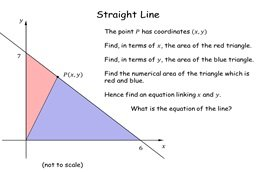A very simple (but illuminating) task where pupils follow simple instructions and generate the equation of a straight line from consideration of areas of triangles.  Each equation is different but easy for the teacher to ascertain the answer by visual inspection.

Worksheet PowerPoint Supporting Materials
Straight Line Straight Line Straight Line (Geogebra)

###### 39. Letter Wheel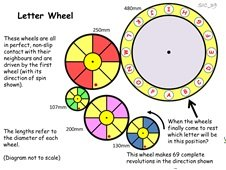Pupils have to work out how much the last wheel of five connected wheels turns when told how much the first wheel turns.  Sixteen different answers but teacher knows each answer from information coded in the worksheet.  Can introduce modulo arithmetic.

Worksheet PowerPoint Supporting Materials
Letter Wheel Letter Wheel Letter Wheel (.sb2)

###### 43. Pick a PolygonPupils discover Pick's Theorem.  Each worksheet has different polygons but polygons allocated to a given letter have the same area.  They are invited to draw more polygons and discover the formula for the area.

Worksheet PowerPoint Supporting Materials
Pick a Polygon Pick a Polygon Proof of Pick

###### 47. How Many Sides?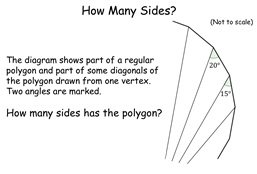On the face of it this looks like a very tricky problem but in fact it is quite straightforward, only requiring knowledge of external angles and the sum of angles in a triangle!  Teacher can easily infer the answer and there is an interesting result at the end.

Worksheet PowerPoint Supporting Materials
How Many Sides? How Many Sides? None

###### 4. Parallelogram in Quadrilateral - Compass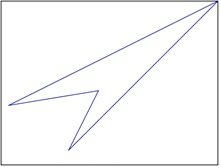Bisect the sides of a quadrilateral to get a parallelogram.  All quadrilaterals are different but all parallelograms are identical.  A compass will be needed to bisect the sides.

Worksheet PowerPoint Supporting Materials

###### 7. Trapezium and DiagonalsWork out the area of two triangles given the area of two others which all fit in a trapezium.  Each problem is different but all the answers are the same.

Worksheet PowerPoint Supporting Materials
Trapezium and Diagonals Trapezium and Diagonals None

###### 35. Strange Guys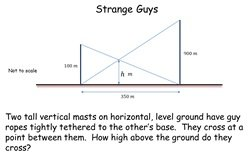Classic problem involving masts and guy ropes solved using the application of similar triangles.  The activity brings out the fact that the distance between the masts is irrelevant to the question posed.  All the answers are the same (90 m).

Worksheet PowerPoint Supporting Materials
Strange Guys Strange Guys None

###### 41. Cross Bear!Three bears start scoffing porridge at different rates and then start scoffing each other's.  Pupils have to work out the individual rates.  Teacher can find the answer encoded in the worksheet by multiplying a number by 10.

Worksheet PowerPoint Supporting Materials
Cross Bear! Cross Bear!
None

###### 42. The Obedient Ray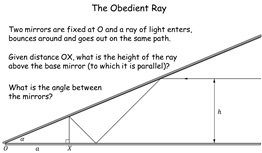A light ray bounces between two mirrors and retraces its steps.  The angle (to be determined) between the mirrors is the same but the height of each ray is different.  Simple geometry yields the answer but pupils are invited to look at a more general case.

Worksheet PowerPoint Supporting Materials
The Obedient Ray The Obedient Ray None

###### 45. Piscatorial Percentages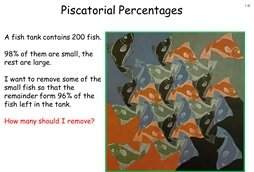A counter-intuitive result involving percentages.  It's a nice little problem to solve algebraically but there is also a very simple way to do it which makes it even more worth doing.  I couldn't resist using some Escher artwork.

Worksheet PowerPoint Supporting Materials
Piscatorial Percentages Piscatorial Percentages
None

###### 16. Angle at Centre, Angle on Arc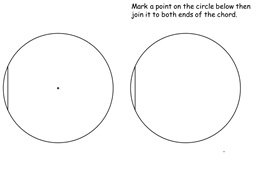Discovery of two circle theorems.  Pupils mark out angles on a chord and cut them out and compare.

Worksheet PowerPoint Supporting Materials
Angle at Centre, Angle on Arc Angle at Centre, Angle on Arc Angle at Centre, Angle on Arc Resources
Angle at Centre, Angle on Arc (Geogebra)

###### 17. Multi-Simultaneous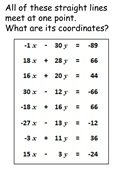Pupils are presented with a number of simultaneous equations to solve.  How quickly will they spot that they only need to select one pair?  How many will select the easiest pair rather than just the first two in the list?.......

Worksheet PowerPoint Supporting Materials
Multi Simultaneous Multi Simultaneous Multi Simultaneous (Excel)

###### 18. Polygon in Annulus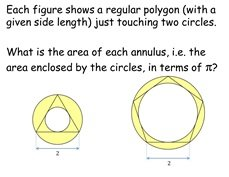Pupils need to work out the area of a couple of rings with different dimensions - but the same area.

Worksheet PowerPoint Supporting Materials
Polygon in Annulus Polygon in Annulus Polygon in Annulus (Geogebra)

###### 21. Leonardo of Pisa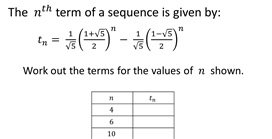Pupils are given the nth term of a sequence and are asked to find specific (non consecutive) terms in the sequence.  It is the Fibonacci sequence.  The task involves surds and indices.  Then they are asked to prove that the ratio of consecutive terms tends to the golden ratio.

Worksheet PowerPoint Supporting Materials
Leonardo of Pisa Leonardo of Pisa Leonardo of Pisa (Excel)

###### 25. Crossed Lines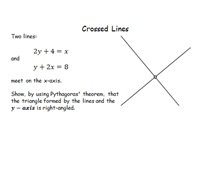This tests the pupils' knowledge of straight line graphs and y=mx+c and the significance of gradients of perpendicular lines.

Worksheet PowerPoint Supporting Materials
Crossed Lines Crossed Lines Crossed Lines (Excel)Problem involving forming and solving a quadratic equation.  Not very hard and won't take long.  Can the pupils work out how all the answers are the same?

Worksheet PowerPoint Supporting Materials

###### 44. Sea Shell Product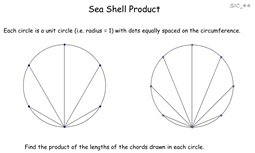Pupils discover an intriguing result (that will be beyond them to prove) - the product of the lengths of the chords equals the number of equally spaced dots on the circle.  Quite simple trigonometry and lengths of sides of triangles.

Worksheet PowerPoint Supporting Materials
Sea Shell Product
Sea Shell Product None

###### 46. Squaring the Rectangle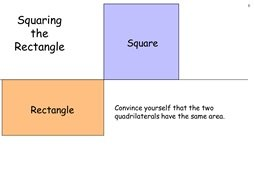Pupils are asked to construct a square with area equal to a given rectangle, using construction methods only.  Gives practice in constructions and application of the intersecting chords theorem.  Leads to determining the square root of a number by construction.

Worksheet PowerPoint Supporting Materials
Squaring the Rectangle
Squaring the Rectangle None

###### 48. Three Circles and a TangentPupils seem to be fazed when they first look at this problem, so it's worth doing.  It only requires Pythagoras' Theorem (like most Sangaku problems).  There is a neat answer to the general case.

Worksheet PowerPoint Supporting Materials
Three Circles and a Tangent
Three Circles and a Tangent None

###### 2. Sine Rule Discovery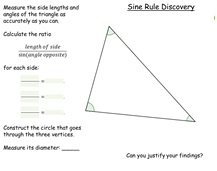Measure the sides and angles and calculate the ratios.  All ratios in the class are the same, yet all of the triangles are different.

Worksheet PowerPoint Supporting Materials
Sine Rule Discovery Sine Rule Discovery None

###### 8. Four Crescents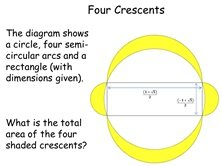Four crescents are drawn around a rectangle.  All rectangles are different and the area of the crescents is equal to the rectangle.  Surd (area = 1) and non-surd (area = 900) versions available.

Worksheet PowerPoint Supporting Materials
Four Crescents Four Crescents Four Crescents 900
Four Crescents (Geogebra)

###### 11. Triangle xy Area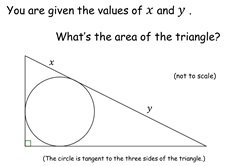Work out the area of a triangle with an inscribed circle.  Answers are all different but easily calculated from the given parameters.

Worksheet PowerPoint Supporting Materials
Triangle xy area Triangle xy area None

###### 34. L-centre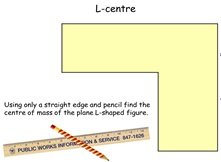Hands-on activity to find the centre of mass of an L-shaped plane figure by construction only.  But there is something special about them all - each centre of mass is at the inner elbow of the L.  Can the (Year 13) pupils work out why this is?

Worksheet PowerPoint Supporting Materials
L-centre L-centre L-centre 1 (Geogebra)
L-centre 2 (Geogebra)

###### 38. Awkward Triangle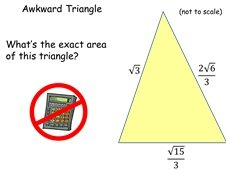Given the side lengths of a scalene triangle (in surd form) can the pupils work out its area?  Tests use of the Cosine Rule and multiplication and simplification of surds.  For interest, Heron's formula is also used to solve the problem..

Worksheet PowerPoint Supporting Materials
Awkward Triangle Awkward Triangle Awkward Triangle (Geogebra)
Awkward Triangle (Excel)

###### 49. What's the Sine Rule Ratio?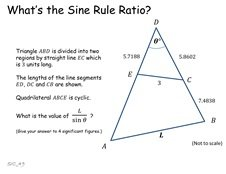Pupils are asked to use their knowledge of sine rule, cosine rule and even a circle theorem or two to determine angles and lengths in a triangle.

Worksheet PowerPoint Supporting Materials
What's the Sine Rule Ratio? What's the Sine Rule Ratio? None

###### 5. A Circle Property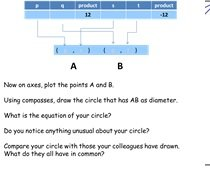RISP 9.  Pupils choose certain values and draw their own circle.  All circles go through the origin.

Worksheet PowerPoint Supporting Materials
A Circle Property A Circle Property A Circle Property (Geogebra)

###### 9. Brackets Out, Brackets In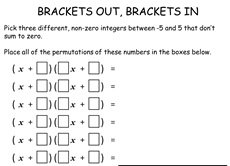RISP 3.  Pupils have to simplify some algebra, that they have generated.  All have the factor (x+1)

Worksheet PowerPoint Supporting Materials
Brackets Out, Brackets In Brackets Out, Brackets In Brackets Out, Brackets In (Excel)

###### 19. Cubic Tangent CirclePupils need to work out the equation of a cubic and draw a tangent at a specific place.  All tangents should go through the first root.

Worksheet PowerPoint Supporting Materials
Cubic Tangent Circle Cubic Tangent Circle None

###### 20. Hyper Parabola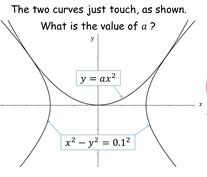Pupils need to find out where a parabola and a hyperbola just touch.

Worksheet PowerPoint Supporting Materials
Hyper Parabola Hyper Parabola Hyper Parabola (Geogebra)

###### 28. Survey Plot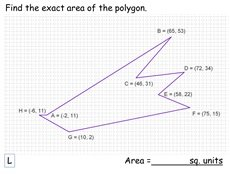Imagine you needed to remove one metre depth of topsoil from a new building plot.  What volume does this represent?  How many wagons will you need, etc?  By approximating this area to a polygon (where you know the coordinates of the vertices) makes the task very easy (even easier with a spreadsheet).

Worksheet PowerPoint Supporting Materials
Survey Plot Survey Plot Survey Plot (Excel)
Survey Plot (Geogebra)

###### 32. How Close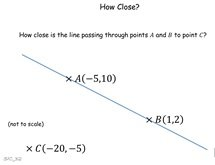A coordinate geometry task.  Pupils have the coordinates of two points on a line.  How close is this line to a third point?  All pairs of coordinates are different but lie on three different lines - but the closest distance is the same for all.

Worksheet PowerPoint Supporting Materials
How Close How Close How Close (Geogebra)
How Close (Excel)

###### 37. Find f(2)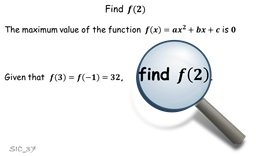A slightly cryptic question based on a quadratic function.  Not too difficult but a little different from the norm.   As an extension you could ask the pupils to come up with their own parameters that give the same solution.

Worksheet PowerPoint Supporting Materials
Find f(2)
Find f(2)
Find f(2) (Geogebra)

###### 13. A Geometric Progression Problem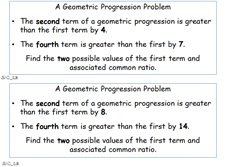From clues given about a geometric progression work out the possible solutions - all common ratios are the same and first terms are five times each other.

Worksheet PowerPoint Supporting Materials
A Geometric Progression Problem A Geometric Progression Problem A Geometric Progress Problem (Excel)

###### 23. A Fractional Sequence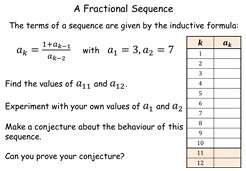An inductive formula is given with each pupil having different starting values.  They have to find the 11th and 12th terms.  The sequences loop (but they don't know that) so they don't need to go that far.  Then, of course, they have to prove it always loops!

Worksheet PowerPoint Supporting Materials
A Fractional Sequence A Fractional Sequence A Fractional Sequence (Excel)

###### 24. Area between ParabolasAn integration activity around the area bounded by two parabolas.  Some pupils are confused  if the area straddles the x-axis.  This activity addresses this.

Worksheet PowerPoint Supporting Materials
Area between Parabolas Area between Parabolas Area between Parabolas (Geogebra)

###### 30. Parabola in ParallelogramGiven certain facts about a parabola the pupils have to determine its equation.  Then they need to integrate the function to work out ratios of areas.  There is a neat solution that makes it very easy to do - which you can show them at the end.  A further extension is to challenge them to do it without integration.

Worksheet PowerPoint Supporting Materials
Parabola in Parallelogram Parabola in Parallelogram Parabola in Parallelogram (Excel)
Parabolas in Parallelogram - no integration (Geogebra)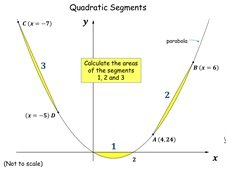Pupils have to work out the area of three segments formed by chords of equal horizontal widths drawn on a parabola.  Interestingly, this area is invariant for a given width.  They discover this and then hopefully prove it.

Worksheet PowerPoint Supporting Materials

###### 6. Annulus Volume of Revolution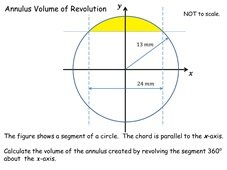Pupils are given different segments of circles and asked to calculate their volumes of revolution.  They all have the same volume.

Worksheet PowerPoint Supporting Materials
Annulus Volume of Revolution Annulus Volume of Revolution Annulus Volume of Revolution (Autograph)
Annulus Volume of Revolution (Excel)

###### 14. Integration Stretch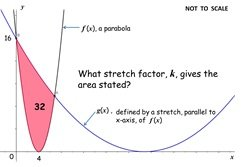A parabola is stretched - calculate the stretch factor to achieve the area bounded between the two.  All parabolas are different but the stretch factors are the same.

Worksheet PowerPoint Supporting Materials
Integration Stretch Integration Stretch Integration Stretch (Excel)
Integration Stretch (Geogebra)

22. The Shortest RaceYou have to run from tree A to tree B whilst touching a fence.  Where should you touch the fence to minimise the distance you have to run?

Worksheet PowerPoint Supporting Materials
The Shortest Race The Shortest Race The Shortest Race (Geogebra)
The Shortest Race (Excel)

###### 27. Skew LinesSkew lines in 3D space in vector form.  How close do they get?

Worksheet PowerPoint Supporting Materials
Skew Lines Skew Lines Skew Lines (Excel)
Skew Lines (Autograph)

###### 29. Volume of Tetrahedron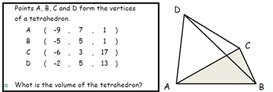Given the 3D coordinates of four points what is the volume of the tetrahedron that is formed by joining them with line segments?  This brings together just about everything they need to know about vectors and so is a good revision task.  Using the triple product to solve it takes some of the fun out (hence suitable up to C4).  Of course, the volumes are all the same.... but why?

Worksheet PowerPoint Supporting Materials
Volume of Tetrahedron Volume of Tetrahedron

Volume of Tetrahedron nets
Volume of Tetrahedron (Excel)

Volume of Tetrahedron net 5 (Geogebra)

###### 26. Shoot the Monkey!Projectiles.  You want to shoot a monkey hanging in a tree.  But he's a cheeky monkey and at the exact moment you fire, he lets go and falls to the ground.  Knowing this, where should you aim to be sure of hitting your target?

Worksheet PowerPoint Supporting Materials
Shoot the Monkey! Shoot the Monkey! Shoot the Monkey! (Geogebra)
Shoot the Money! (Excel)

###### 33. Target Range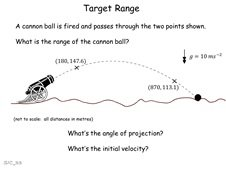Simple projectiles problem.  Given two points on a trajectory the pupils need to ascertain range, angle of elevation and muzzle velocity.  A good test to see if they can remember how to do it from first principles.

Worksheet PowerPoint Supporting Materials
Target Range Target Range
Target Range (Excel)

###### 34. L-centreHands-on activity to find the centre of mass of an L-shaped plane figure by construction only.  But there is something special about them all - each centre of mass is at the inner elbow of the L.  Can the (Year 13) pupils work out why this is?

Worksheet PowerPoint Supporting Materials
L-centre L-centre L-centre 1 (Geogebra)
L-centre 2 (Geogebra)

###### 10. Magic Bag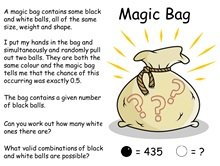nRich.  An algebra task thinly disguised as a probability question.  The pupils have to determine the number of white balls in a bag.  There are two answers!  Triangular numbers are involved.

Worksheet PowerPoint Supporting Materials
Magic Bag Magic Bag None

###### 50. Misprints do Hapen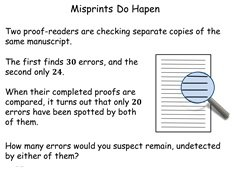Two proof-readers find errors in the same manuscript - but not all the same ones.  How many are likely to have been missed by both?   This problem seems intractable at first but is quite straightforward if you keep a clear head.  The pupils should be challenged to create a new worksheet with the same answer, 2.

Worksheet PowerPoint Supporting Materials
Misprints do Hapen Misprints do Hapen None